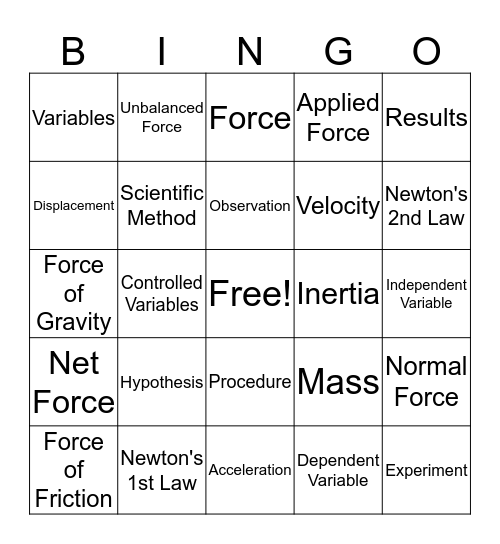# Vocab BingoThis bingo card has a free space and 27 words: Mass, Speed, Velocity, Acceleration, Displacement, Force, Unbalanced Force, Net Force, Inertia, Normal Force, Force of Gravity, Applied Force, Force of Friction, Newton's 1st Law, Newton's 2nd Law, Newton's 3rd Law, Scientific Method, Observation, Hypothesis, Variables, Independent Variable, Dependent Variable, Controlled Variables, Experiment, Procedure, Results and Data.

## Play Online